# KSEEB Solutions for Class 5 Maths Chapter 9 Perimeter and Area

Students can Download Maths Chapter 9 Perimeter and Area Questions and Answers, Summary, Notes Pdf, KSEEB Solutions for Class 5 Maths helps you to revise the complete Karnataka State Board Syllabus and score more marks in your examinations.

## Karnataka State Syllabus Class 5 Maths Chapter 9 Perimeter and Area

### KSEEB Class 5 Maths Perimeter and Area Ex 9.1

Question 1.
What are two-dimensional figures called?
Plane figures

Question 2.
Name the two dimensions of a rectangle.Question 3.
How many pairs of opposite sides are there in a rectangle?
Two pairs

Question 4.
How are the opposite sides in a rectangle?
Equal

Question 5.
How many times is the perimeter of a rectangle to the sum of its length and breadth?
TwiceII. Find the perimeter of the following pictures:Perimeter of Rectangle = (21 + 2b)
= (2 × 70 + 2 × 90)
= 140 + 180
= 320 cm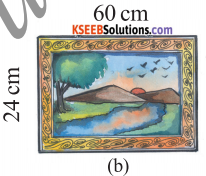Perimeter of Rectangle = (21 + 2b)
= (2 × 60 + 2 × 24)
= 120 + 48
= 168 cm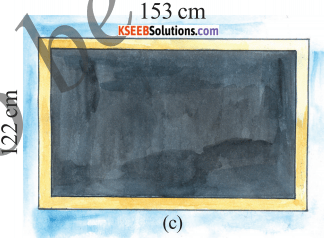Perimeter of Rectangle = (21 + 2b)
= (2 × 153 + 2 × 122)
= 306 + 244
= 550 cm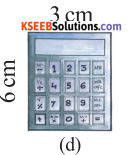Perimeter of Rectangle = (21 + 2b) units
= (2 × 3 + 2 × 6)
= 6+12
= 18cmPerimeter of Rectangle
= (21 + 2b)
= (2 × 92 + 2 × 80)
= (184 + 160)
= 344 cmIII. The length and breadth of rectangles are given below. Find their perimeters.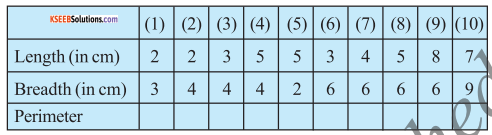1) The Perimeter of Rectangle = (21 + 2b)
= (2 × 2 + 2 × 3)
= 4 + 6
= 10 cm2) The Perimeter of Rectangle = (21 + 2b)
= (2 × 2 + 2 × 4)
= 4 + 8
= 12 cm

3) The Perimeter of Rectangle = (21 + 2b)
= (2 × 3 + 2 × 4)
= 6 + 8
= 14 cm

4) The Perimeter of Rectangle = (21 + 2b)
= (2 × 5 + 2 × 4)
= 10 + 8
= 18 cm5) The Perimeter of Rectangle = (21 + 2b)
= (2× 5 + 2 × 2)
= 10 + 4
= 14 cm

6) The Perimeter of Rectangle = (21 + 2b)
= (2 × 3 + 2 × 6)
= 6 + 12
= 18 cm

7) The Perimeter of Rectangle = (21 + 2b)
= (2 × 4 + 2 × 6)
= 8 + 12
= 20 cm8) The Perimeter of Rectangle = (21 + 2b)
= (2 × 5 + 2 × 6)
= (10 + 12)
= 22 cm

9) The Perimeter of Rectangle = (21 + 2b)
= (2 × 8 + 2 × 6)
= 16 + 12
= 28 cm

10) The Perimeter of Rectangle = (21 + 2b)
= (2 × 7 + 2 × 9)
= 14 + 18
= 32 cm

IV. Solve the following:

Question 1.
A rectangular room measure 6m in length and 4m in breadth. Find the perimeter of the room.
Length = 6m
The Perimeter of a room = (21 + 2b) units
= (2 × 6 + 2 × 4)
= 12 + 8
= 20 metersQuestion 2.
A rectangular field has a length of 150m and breadth 120m. Find the perimeter of the field.
The Perimeter of the field = (21 + 2b) units
= (2 × 150 + 2 × 120)
= 300 + 240
= 540 metersQuestion 3.
A rectangular garden measures 80m in length and 50m in breadth. Find its perimeter. If the garden has to be fenced 5 rounds with barbed wire, what is the length of the wire required?
The Perimeter of Rectangular Garden
= (21 + 2b) units
= (2 × 80 + 2 × 50)
= 160 + 100 = 260 meters
The Garden has to be fenered 5rounds with barbed wire = 260 × 5
= 1300 metresQuestion 4.
An auditorium measures 80m in length and 30 min breadth. If the walls of the auditorium have to be decorated with colored buntings 4 times, find the length of buntings required. If the cost of 1m of buntings is Rs. 15, what is the total cost of the buntings used to decorate the auditorium?
The Perimeter of Auditorium = (21 + 2b) units = (2 × 80 + 2 × 30)
= 160 + 60
= 220 metres
Auditorium walls have to be decorated with colour buntings = 220 × 4 = 880 metres The cost of the buntings used to decorate the auditorium = 880 × 15 = 13,220
The cost of 1 metre of bunting = Rs 15Question 5.
Srilatha, during her morning walk, goes round a rectangular park 3 times. If the length and breadth of the park are 320 m and 210 m respectively, calculate the distance she has covered.
The Perimeter of Rectangular Parks
= (21 + 2b) units
= (2 × 320 + 2 × 210)
= 640 + 420
= 1060 metres
Srilatha walks round Rectangular park
= 3 times
= 1060 × 3
= 3180 metres
Srilatha walks 3180 metres of distance

### KSEEB Class 5 Maths Perimeter and Area Ex 9.2

Question 1.
Mention the two dimensions of a square.
Length × width

Question 2.
How many equal sides are there in a square?
4Question 3.
How many times is the perimeter of a square to its length?
Four times

Question 4.
What is the perimeter of a square of length 5 cm?
The perimeter of a square
= 4 × 1
= 4 × 5
= 20 cmII. The rectangle given below are divided into squares of unit length. Find their areas:The perimeter of a square
= 4 × 1
= 4 × 14
= 56 cm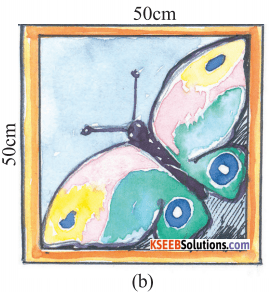The perimeter of a square
= 4 × 1
= 4 × 50
= 200cmThe perimeter of a square
= 4 × 1
= 4 × 52
= 208 cmThe perimeter of a square
= 4 × 1
= 4 × 45
= 180 cm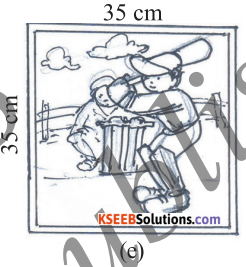The perimeter of a square
= 4 × 1
= 4 × 35
= 120 cmIII. Lengths of the squares are given in the following table. Find their perimeters.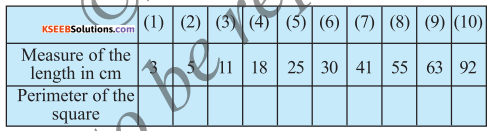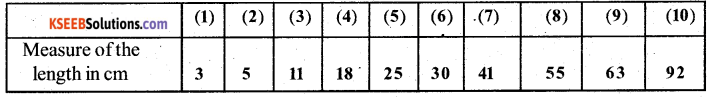a) The perimeter o f a square = 4 × 1 = 4 × 3 = 12 cm
b) The perimeter of a square = 4 × 1 = 4 × 5 = 20 cm
c) The perimeter of a square = 4 × 1 = 4 × 11 = 44 cm
d) The perimeter of a square = 4 × 18 = 72 cm
e) The perimeter of a square = 4 × 25 = 100 cm
f) The perimeter of a square = 4 × 30 = 120 cm
g) The perimeter of a square = 4 × 41 = 164 cm
i) The perimeter of a square = 4 × 55 = 220 cm
j) The perimeter of a square = 4 × 63 = 252 cm
k) The perimeter of a square = 4 × 92 = 368 cmIV. Solve the following problems:

Question 1.
The length of a square room is 15m. Find its perimeter.
The perimeter of a square room
= 4 × 1
= 4 × 15
= 60 meterQuestion 2.
Rama, runs 4 times around a square park of length 85 m. What is the total distance he covers?
The perimeter of a square park
= 4 × 1
= 4 × 85
= 340 mt
Rama runs 4 times around a park
= 340 × 4
= 1360mt

Question 3.
The length of a square room is 16m. The walls of the room should be tied with colored buntings 4 times. Find the total length of buntings required.
The perimeter of a square room
= 4 × 1
= 4 × 6
= 64 cm

The room should be tied with colored buntings 4 times
= 64 × 4
= 256 mtQuestion 4.
The length of a square ground is 70m. Find its perimeter. (Additional Que)
The perimeter of a square ground
= 4 × 1
= 4 × 70
= 280 mt

Question 5.
The length of a square playground is 30m. Find its perimeter. (Additional Question)
The perimeter of a square playground
= 4 × 30
= 120 mt

### KSEEB Class 5 Maths Perimeter and Area Ex 9.3

Question 1.
What is the unit of area?
Square units.

Question 2.
What is the space within the boundary of a plane figure called?
Area

Question 3.
What is the area of a unit square?
Unit squareII. The rectangle given below are divided into squares of unit length. Find their areas: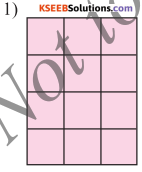Area of Rectangle
= (1 × b) units
= 3 × 4
= 12 sq.units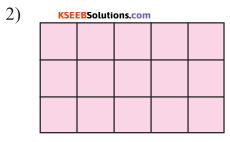Area of Rectangle
= (1 × b) units
= 5 × 3
= 15 sq.units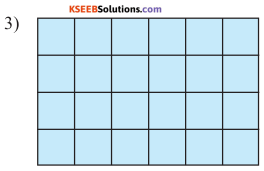Area of Rectangle
= (1 × b) units
= 6 × 4
= 24 sq.units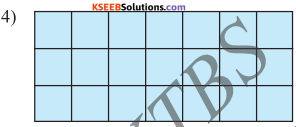Area of Rectangle
= (1 × b) units
= 7 × 3
= 21 sq.unitsArea of Rectangle
= (1 × b) units
= 8 × 4 = 32 sq.unitsIII. The length and breadth of the rectangles are given below. Calculate their areas: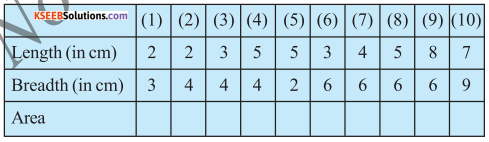(1) Area of Reactangle
= 1 × b
= 2 × 3
= 6 sq.cm(2) Area of Reactangle
= 1 × b
= 2 × 4
= 8 sq.cm

(3) Area of Reactangle
= 1 × b
= 3 × 4
= 12 sq.cm

(4) Area ofRectangle
= 1 × b
= 5 × 4
= 20 Sq.cm(5) Area ofRectangle
= 1 × b
= 5 × 2
= 10 Sq.cm

(6) Area of Rectangle
= 1 × b
= 3 × 6
= 18 Sq.cm

(7) Area of Rectangle
= 1 × b
= 4 × 6
= 24 Sq.cm(8) Area ofRectangle
= 1 × b
= 5 × 6 = 30 Sq.cm

(9) Area of Rectangle
= 1 × b
= 8 × 6
= 48 Sq.cm

(10) Area ofRectangle
= 1 × b
= 7 × 9
= 63 Sq.cmIV. Find the area of the figures given below:Area ofRectangle
= 1 × b
= 70 × 90
= 6300 Sq.cmArea of Rectangle
= 1 × b
= 60 × 20
= 1200 Sq.cm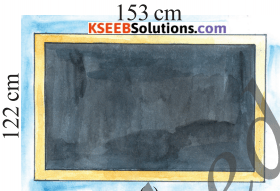Area of Rectangle
= 1 × b
= 153 × 122
= 18,666 Sq.cmArea of Rectangle
= 1 × b
= 3 × 6
= 18 Sq.cmArea of Rectangle
= 1 × b
= 92 × 80
= 7,360 Sq.cmV. Solve the following problems:

Question 1.
A farmer has a rectangular land length 250m and breadth 180m. Find the area of the land.
Area of the land
= 1 × b
= 250 × 180
= 45,000 Sq.cm

Question 2.
A carpet is needed to cover the entire area of a room. If the length of the room is 16m and breadth 5m, find the area of the carpet required.
Area of the carpet
= 1 × b
= 16 × 5
= 80 Sq.cmQuestion 3.
An auditorium measures 25m in length and 18m in breadth. How many slabs of stones of 3m × 1m are required to cover the floor of the auditorium?
Area of Auditorium
= 1 × b
= 25 × 18
= 450 Sq.cm

The size of the slab stane
= 3m × 1m
= 3m

The number of slab stanes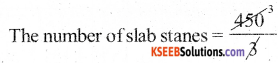= 150Question 4.
A rectangular plot’s length is 25m and breadth is 15m. Find the area of the plot. If 1 square meter of the plot costs Rs. 250, what is the total value of the plot?
Area of the plot = 1 × b
= 25 × 15
= 375 Sq.cm

1 Sq.mt of the plot Rs 250
375 Sq.mt of the plot = 375 × 250
= 93,750Question 5.
A rectangular room’s length is 20m and breadth is 11m. How many tiles of 2m × 1m are required to cover the floor of the room?
Area of room
= 1 × b
= 20 × 11
= 220 Sq.cm

The number of tiles to cover the floor of the room
= 2m × 1m
= 2m### KSEEB Class 5 Maths Perimeter and Area Ex 9.4

Question 1.
What is the total space within the boundary of a square called?
AreaQuestion 2.
What is the unit of area?
(1 × 1)

Question 3.
What is the area of a square?
Sq.unitII. Find the area of the figures given below:Area of a square
= 1 × 1
= 52 × 52
= 2,704 Sq.cm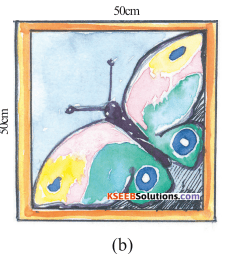Area of a square
= 1 × 1
= 50 × 50
= 2,500 Sq.cm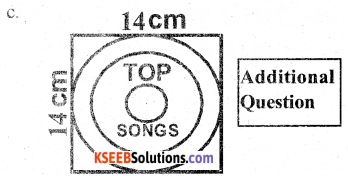Area of a square
= 1 × 1
= 14 × 14
= 136 Sq.cmArea of a square
= 1 × 1
= 45 × 45
= 2,025 Sq.cm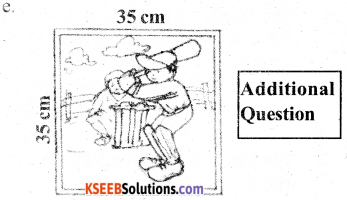Area of a square
= 1 × 1
= 35 × 35
= 1,225 Sq.cmIII. The measure of one side of the squares are given below. Find their areas.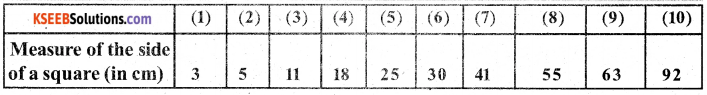(1) Area of a Square
= 1 × 1
= 3 × 3 Sq.cm

(2) Area of a Square
= 1 × 1
= 5 × 5
= 25 Sq.cm(3) Area of a Square
= 1 × 1
= 11 × 11
= 121 Sq.cm

(4) Area of a Square
= 1 × 1
= 18 × 18
= 324 Sq.cm

(5) Area of a Square
= 1 × 1
= 25 × 25
= 625 Sq.cm(6) Area of a Square
= 1 × 1
= 30 × 30
= 900 Sq.cm

(7) Area of a Square
= 1 × 1
= 41 × 41
= 1,681 Sq.cm

(8) Area ofa Square
= 1 × 1
= 55 × 55
= 3,025 Sq.cm(9) Area of a Square
= 1 × 1
= 63 × 63
= 3,969 Sq.cm

(10) Area ofa Square
= 1 × 1
= 92 × 92
= 8464 Sq.cmIV. Solve the following problems:

Question 1.
The length of a square room is 6m. What is the area of the floor of the room?
Area of the floor of the room
= 1 × 1
= 6 × 6
= 36 sq.mt

Question 2.
The length of a square paper is 21 cm. What is its area?
Area of a square paper
= 1 × 1
= 21 × 21
= 441 sq.cmQuestion 3.
The length of a square canvas cloth is 15m. Find the area of the cloth?
Area of the cloth
= 1 × 1
= 15 × 15
= 225 Sq.mt

Question 4.
The length of a square room is 8m. How many granite stones of 2m × 1m are required to cover the floor of the room?
Area of a square room
= 1 × 1
= 8 × 8
= 64 Sq.mt
The number of granites
= 2m × 1m
= 2m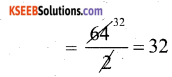Question 5.
The length of a square room is 400cm. How many square tiles of 10cm in length, are required to cover the floor of the room.
Area of a square
= 1 × 1
= 400 × 400
= 1600 sq.cm
The length of a square tile = 10cm
The number of tiles to cover 1,60,000Area of tiles = 10 × 10
= 100 sq.cmerror: Content is protected !!